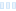Hello all,

I'm finally checking out UF6, and the deep zoom is amazingly fast! I've run into a question, though. In a lot of my older fractals, I used Lyapunov coloring layers in deep(ish) zooms. When I load some of those old parameters into UF6, it looks like Lyapunov stops working at the deeper levels of magnification. I used to fix that by manually adjusting the Additional Precision. Can anyone tell me which settings to tweak in the new version? Or is there something about the new, faster calculation method that's just incompatible with the Lyapunov coloring method?

Here's a parameter set as an example. If you paste it into UF6, there are solid-colored places where the coloring isn't applied the way I'm used to. In UF5, the Lyapunov texture fills those in.

`lyapunovGlitch {fractal:  title="lyapunov glitch" width=660 height=510 layers=2  credits="Morgen Bell;6/26/2019"layer:  caption="lyapunov" opacity=100 method=multipass transparent=yesmapping:  center=0.308437706756697737998947674/0.0252620075616027513364048156  magn=2.3222389E18 angle=13.5238formula:  maxiter=50182 adjust=yes filename="Standard.ufm" entry="Mandelbrot"  p_start=0/0 p_power=2/0 p_bailout=1e20inside:  transfer=none offset=88 solid=0 filename="Standard.ucl"  entry="Default"outside:  density=4 transfer=linear filename="dmj.ucl" entry="dmj-Lyapunov"  p_trackvariable="real / imag" p_negative="absolute value"  p_power=2.0 p_bailout=1E20 p_smooth=yesgradient:  smooth=yes rotation=-33 index=60 color=8471413 index=168  color=2799072 index=252 color=10116202 index=-25 color=12290633opacity:  smooth=no index=0 opacity=255layer:  caption="smooth" opacity=100 method=multipass transparent=yesmapping:  center=0.308437706756697737998947674/0.0252620075616027513364048156  magn=2.3222389E18 angle=13.5238formula:  maxiter=50182 adjust=yes filename="Standard.ufm" entry="Mandelbrot"  p_start=0/0 p_power=2/0 p_bailout=1000inside:  transfer=none offset=88 solid=0 filename="Standard.ucl"  entry="Default"outside:  transfer=sqrt filename="Standard.ucl" entry="Smooth" p_power=2/0  p_bailout=128.0gradient:  smooth=yes rotation=-26 index=128 color=5921370 index=-215  color=16777215 index=-70 color=0opacity:  smooth=no index=0 opacity=255}`

Hello all, I&#039;m finally checking out UF6, and the deep zoom is amazingly fast! I&#039;ve run into a question, though. In a lot of my older fractals, I used Lyapunov coloring layers in deep(ish) zooms. When I load some of those old parameters into UF6, it looks like Lyapunov stops working at the deeper levels of magnification. I used to fix that by manually adjusting the Additional Precision. Can anyone tell me which settings to tweak in the new version? Or is there something about the new, faster calculation method that&#039;s just incompatible with the Lyapunov coloring method? Here&#039;s a parameter set as an example. If you paste it into UF6, there are solid-colored places where the coloring isn&#039;t applied the way I&#039;m used to. In UF5, the Lyapunov texture fills those in. lyapunovGlitch { fractal: title=&quot;lyapunov glitch&quot; width=660 height=510 layers=2 credits=&quot;Morgen Bell;6/26/2019&quot; layer: caption=&quot;lyapunov&quot; opacity=100 method=multipass transparent=yes mapping: center=0.308437706756697737998947674/0.0252620075616027513364048156 magn=2.3222389E18 angle=13.5238 formula: maxiter=50182 adjust=yes filename=&quot;Standard.ufm&quot; entry=&quot;Mandelbrot&quot; p_start=0/0 p_power=2/0 p_bailout=1e20 inside: transfer=none offset=88 solid=0 filename=&quot;Standard.ucl&quot; entry=&quot;Default&quot; outside: density=4 transfer=linear filename=&quot;dmj.ucl&quot; entry=&quot;dmj-Lyapunov&quot; p_trackvariable=&quot;real / imag&quot; p_negative=&quot;absolute value&quot; p_power=2.0 p_bailout=1E20 p_smooth=yes gradient: smooth=yes rotation=-33 index=60 color=8471413 index=168 color=2799072 index=252 color=10116202 index=-25 color=12290633 opacity: smooth=no index=0 opacity=255 layer: caption=&quot;smooth&quot; opacity=100 method=multipass transparent=yes mapping: center=0.308437706756697737998947674/0.0252620075616027513364048156 magn=2.3222389E18 angle=13.5238 formula: maxiter=50182 adjust=yes filename=&quot;Standard.ufm&quot; entry=&quot;Mandelbrot&quot; p_start=0/0 p_power=2/0 p_bailout=1000 inside: transfer=none offset=88 solid=0 filename=&quot;Standard.ucl&quot; entry=&quot;Default&quot; outside: transfer=sqrt filename=&quot;Standard.ucl&quot; entry=&quot;Smooth&quot; p_power=2/0 p_bailout=128.0 gradient: smooth=yes rotation=-26 index=128 color=5921370 index=-215 color=16777215 index=-70 color=0 opacity: smooth=no index=0 opacity=255 }

0

The Lyapunov coloring clearly needs access to high-precision values of #z, and currently in UF6 it only gets the values in double precision. This works for many coloring algorithms but not for this one.

I don't have a good solution for this at the moment, but I'll think about it and do something for the next update release. This will be a way to go back to the previous behavior, so you'll get slow (but correct) arbitrary precision math again.

The Lyapunov coloring clearly needs access to high-precision values of #z, and currently in UF6 it only gets the values in double precision. This works for many coloring algorithms but not for this one. I don&#039;t have a good solution for this at the moment, but I&#039;ll think about it and do something for the next update release. This will be a way to go back to the previous behavior, so you&#039;ll get slow (but correct) arbitrary precision math again.

Ultra Fractal author

edited Jul 2 '19 at 7:59 pm

0
156
views
1
replies
2
followers
live preview
Enter at least 10 characters.
WARNING: You mentioned %MENTIONS%, but they cannot see this message and will not be notifiedSaving...
Saved
All posts under this topic will be deleted ?
Pending draft ... Click to resume editing